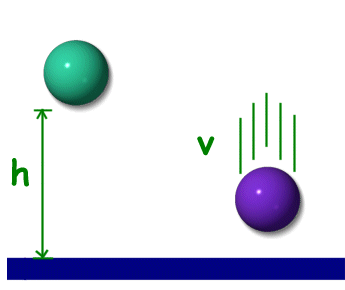Science >> Physics for Kids

# Physics for Kids

## Kinetic Energy

What is kinetic energy?

Kinetic energy is the energy an object has due to its motion. As long as an object is moving at the same velocity, it will maintain the same kinetic energy.

The kinetic energy of an object is calculated from the velocity and the mass of the object. As you can see from the equation below, the velocity is squared and can have a significant impact on the kinetic energy.

Here is the equation for calculating kinetic energy (KE):

KE = 1/2 * m * v2

where m = mass and v = velocity

How to Measure Kinetic Energy

The standard unit for kinetic energy is the joule (J). The joule is the standard unit for energy in general. Other units for energy include the newton-meter (Nm) and the kilogram meter squared over seconds squared (kg m2/s2).

Kinetic energy is a scalar quantity, which means it only has a magnitude and not a direction. It is not a vector.

How is it different from potential energy?

Kinetic energy is due to an object's motion while potential energy is due to an object's position or state. When you calculate an object's kinetic energy, its velocity is an important factor. Velocity, however, has nothing to do with an object's potential energy.The green ball has potential energy due
to its height. The purple ball has kinetic
energy due to its velocity.

Example Using A Roller Coaster

One way to think of potential and kinetic energy is to picture a car on a roller coaster. As the car travels up the coaster it is gaining potential energy. It has the most potential energy at the top of the coaster. As the car travels down the coaster, it gains speed and kinetic energy. At the same time it is gaining kinetic energy, it is losing potential energy. At the bottom of the coaster the car has the most speed and the most kinetic energy, but also the least potential energy.

Example problems:

1. A car and a bicycle are traveling at the same speed, which has the most kinetic energy?

The car does because it has more mass.

2. A ball weighs around 1 kg and is traveling at 20 meters per second, what is its kinetic energy?

KE = 1/2 * m * v2
KE = 1/2 * 1kg * (20 m /s)2
KE = 200 J

3. A boy weighs 50 kg and is running 3 meters per second, what is his kinetic energy?

KE = 1/2 * m * v2
KE = 1/2 * 50 kg * (3 m/s)2
KE = 225 J

• If you double the mass of an object, you double the kinetic energy.
• If you double the speed of an object, the kinetic energy increases by four times.
• The word "kinetic" comes from the Greek word "kinesis" which means motion.
• Kinetic energy can be passed from one object to another in the form of a collision.
• The term "kinetic energy" was first coined by mathematician and physicist Lord Kelvin.
Activities

More Physics Subjects on Motion, Work, and Energy

Science >> Physics for Kids